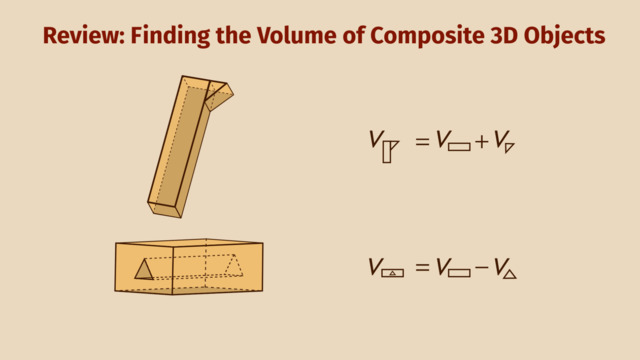# Finding the Volume of Composite 3D ObjectsRating

Be the first to give a rating!

The authorsChris S.

## Basics on the topicFinding the Volume of Composite 3D Objects

After this lesson you will be able to use addition and subtraction to find the volume of solids composed of multiple prisms.

The lesson begins by observing that composite objects can be broken down into smaller pieces. It leads to finding the volume of a rectangular and triangular prism. It concludes with using subtraction to calculate the volume of a mold.

Learn about the volume of composite 3D objects by helping Wolfgang find how much silver he needs to make one of his anti-werewolf tools!

This video includes key concepts, notation, and vocabulary such as: composite object (a 3D solid composed of one or more rectangular prisms).

Before watching this video, you should already be familiar with the concept of volume, calculating volume of a prism, and volume formulas.

After watching this video, you will be prepared to learn how to calculate the volume of composite figures involving different types of prisms.

Common Core Standard(s) in focus: 7.G.B.2 A video intended for math students in the 7th grade Recommended for students who are 12-13 years old

### TranscriptFinding the Volume of Composite 3D Objects

The werewolf is out again, frightening the villagers with his howling. The villagers protect themselves with the silver tools their blacksmith, Wolfgang, forges and sells to them. In order to keep up with demand, Wolfgang has to find the volume of composite 3D objects. Let's find out how much silver Wolfgang needs to make one of his silver tools. It is composed of a rectangular prism and a triangular prism. We can find the volume of the tool, by finding the volume of the rectangular prism and the triangular prism separately, and then adding their volumes together, to get the total volume. Let's start with the rectangular prism. It's dimensions are 20 by 4 by 5 centimeters. Remember, the volume of a rectangular prism is the area of the base times the height. The base is a rectangle so it's area is the length times the width that's 20 times 4 times the height 5. So the volume of the rectangular prism is 400 cubic centimeters. Let's plug that into our equation for the total volume. Now let's look at the triangular prism. It's dimensions are 3 centimeters by 4 centimeters by 5 centimeters. Its volume is also the area of the base times the height. Although, a triangular prism has a triangular base. How do we find the area of a triangle? The area of a triangle is one-half base, times the height. Let's take the 3 centimeter side as the base of the base! Then the height is 4 centimeters. The area of the base in this case is one-half, 3 times 4. That's 6 centimeters. The volume is the area of this base times the height of 5. So that's 6 times 5 or 30 cubic centimeters. Let's plug that into our equation for the total volume. Altogether, the volume of the mace is 400 plus 30, or 430 centimeters cubed. That's a lot of silver! Wolfgang crafts the mace with meticulous care for sale to another frightened villager. To make his newest anti-werewolf device, Wolfgang needs to first make a new mold out of iron. Here's the plan for the mold. Wolfgang needs to find the volume of the mold, so he knows how much iron he needs to make it. To find the volume of the mold, we calculate the volume of the rectangular prism and the triangular prism separately, and then we subtract the volume of the triangular prism from the volume of the rectangular prism. Let's first find the volume of the rectangular prism. The dimensions of the prism are 30 centimeters by 15 by 10. Remember, the volume is length times width times height, so that's 30 times 15 times 10 or forty-five hundred cubic centimeters. Let's plug that into our equation for the total volume. Now, the triangular prism. Remember, the volume of a triangular prism is the area of the base times the height. The triangular base has a base length of 5 centimeters and a height of 4 centimeters. We know that the formula for the area of a triangle is one-half, base times height. So the area of this triangle is one-half times 5 times 4 or 10. The height of the prism is 30 so the volume is the area of this base times 30. So that's 10 times 30, or 300 cubic centimeters. We find the volume of the iron mold by subtracting this from the volume of the rectangular prism. That's forty-five hundred minus 300, which is forty-two hundred cubic centimeters. With his new mold made, he begins to craft his next best-seller. While Wolfgang is crafting, let's review how we calculated the volume of composite objects. First, we observed that some composite objects can be broken down into smaller pieces whose volume is easier to calculate. The first tool was composed of a rectangular prism and a triangular prism. We calculated those volumes individually, then added them. Calculating the volume of the mold was a different story. In this case, we calculated the volume of a large rectangular prism, and subtracted the volume of the empty space inside, a triangular prism. The trick is visualizing the shapes that make up a composite figure, calculating their individual volumes then adding or subtracting as needed. Wolfgang sold all his anti-werewolf gear, but that night, the werewolf returns again! His howls strike terror in the hearts of the villagers. Wolfgang will have to sell them even more tools! What? Not cool, Howlin' Wolfgang. You're a cheat in wolf's clothing!